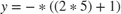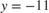Scalar Expansion with the Coulomb and Viscous Friction Block

This example shows a model with a scalar input to a Coulomb & Viscous Friction block that uses scalar expansion to output a vector.Double click the friction block to see the parameters. Coefficient of viscous friction (Gain) is a scalar value 2, but Coulomb friction value (Offset) is a vector value [1 3 2 0] . Therefore, the block uses element-wise scalar expansion to compute the output.

Each output is calculated using this formula.For example, the first offset 1 is calculated as follows.If the dimensions for the input and Offset are the same, then no expansion is necessary.